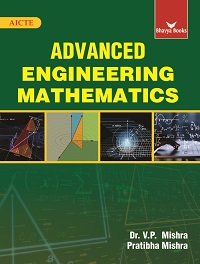•## Advanced Engineering Mathematics (Bhavya Books)

In Stock
ISBN 978-93-83992-34-8 Dr. V.P. Mishra, Dr. Jyoti Sinha, Pratibha Mishra Bhavya Books 2nd 2019 2018 1250 Print In Stock
Rs 795.00Rs 716.00

#### CONTENTS

• Successive Differentiation, Mean Value Theorems and Indeterminate Forms • Applications of Differentiation: Expansion of Functions and Approximations, Curve Tracing, Curvature, Evolute and Involute, Maxima Minima • Sequences and Series: Convergence and Divergence of Infinite Series, Conditional and Absolute Convergence • Definite Integrals, Reduction Formulae, Beta and Gamma Functions • Applications of IntegrationArea, Length, Volume, Surface Area • Fourier Series, Half-Range Series • Partial Differentiation, Euler's Theorem, Change of Variables, Expansion of Functions, Approximation, Jawbians, Maxima-Minima • Matrices, Determinants, Inverse and Rank of Matrix, Hermitian and Skew-Hermitian Matrices • Consistency of System of Equations, Eigen Values, Eigen Vectors, Diagonalizing a Matrix, Vector Spaces • Improper integrals Differentiation under Integral SignLebneitz Rule, Multiple Lebneitz, Double and Triple Integrals and Applications • Vector Calculus: Gradient, Divergence, Curl, Line Integral, Surface Integral, Green's Lemma, Stokes Theorem Gauss Divergence Theorem • Ordinary Differential Equations of Order One: Linear, Exact, Equations of First Order but not of First Degree • Linear Differential Equations of Higher Order, Method of Variation of Parameters and Applications Cauchy's Form • Series Solution, Bessel's Differential Equation and Legendre's Differential Equation • Complex Number System: Complex Functions, Differentiation and Analyticity, Conformal and Bilinear Mapping • Complex Integration: Taylor and Laurent's Series, Residue Theorem, Evaluation of Real Integrals • Laplace Transformation and Its Inverse, Convolution, Solving Differential Equations by L' Transform Unit Step Function, Impulse Function • Partial Differential Equations and Solution to First Order Equations • Partial Differential Equations of Higher Order and Applications • Fourier Transforms and their Applications • Difference Equations, Z-transform and Solution to Difference Equations by Z-transform • Numerical Solution to Equations in One Variable and Simultaneous Equations • Finite Differences, Divided Differences and Interpolation • Numerical Differentiation and Numerical Integration • Numerical Solution to Ordinary Differential Equations of Order One and Order Two and Numerical Solution to Partial Differential Equations • Basic Statistics and Applied Statistics • Theory of Probability • Discrete Probability Distributions Binomial and Poisson and Continuous Probability Distribution Normal • Sampling, Tests of Significance for Large Samples and for small samples • Sets, Relations, Functions, Partial and Total Order Relation, Lattice, Boolean Algebra and Algebraic Structures • Mathematical Logic, Counting Principles and Recurrence Relations • Graph Theory • Answers and Hints to the Problems in Exercises

• Author : Dr. V.P. Mishra, Dr. Jyoti Sinha, Pratibha Mishra
• Publisher : Bhavya Books
• Edition : 2nd 2019
• Publishing Year : 2018
• Total Pages : 1250